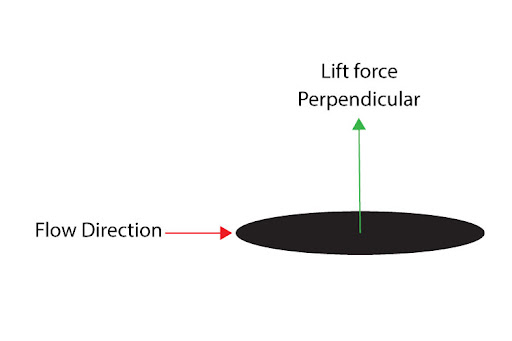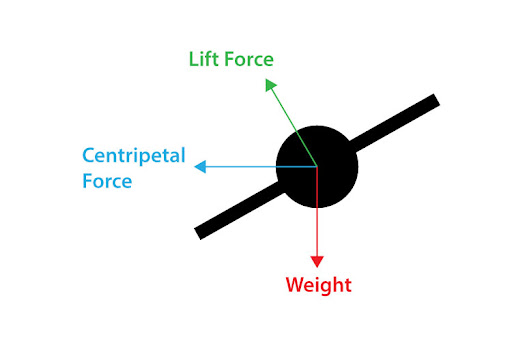# "A plane is entering a turn, identify the main forces acting on it (including central forces)"

A: There are three main forces acting on a plane that has just begun to enter a turn:
1.  Weight
Weight is the gravitational force acting on the plane by virtue of its mass. It is directed vertically downwards.
2.  Lift force
The lift force is the force acting perpendicular to the incoming direction of airflow. For a plane, the airflow is directed against the plane wings, therefore the lift force is directed normal to the plane wings.3.  Centripetal force
This force is what we call a center-seeking force - i.e. it is the force directed towards the center of the circular path, responsible for its circular motion.
All three forces can be drawn as follows: